# KS1 English Quiz - Alphabet - Upper and Lower Case Letters 2 (Questions)

This quiz asks questions on upper and lower case Letters and is for the first term in Year 1 (KS1). This activity will aid both handwriting and sentence level as set out in the National Curriculum.

By KS1, children need to know the alphabet and also need to be able to form each letter correctly by using either upper or lower case. This quiz asks the children to match the upper and lower case letters, reminding them of their varying formation.

This game will assist their sentence, English and literacy level skills.

We begin every sentence and name with a capital letter; all the other letters are lower case. The alphabet has 26 upper case letters (ABC) and lower case letters (abc). Every time you write your name, you begin with an upper case letter and the rest are lower case. See how many letters you remember.

To see a larger image, click on the picture.

1. Egg begins with ‘E’ but should only be capitalized when at the beginning of a sentence. Which of these is the lower case letter for ‘E’?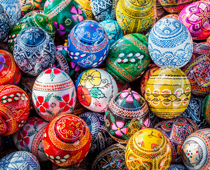[ ] f [ ] e [ ] a [ ] n
2. Jump begins with a capital 'J' when at the beginning of a sentence. Which of these is the lower case letter for ‘J’?[ ] l [ ] j [ ] t [ ] s
3. The word nibble begins with ‘n’. Which of these is the upper case letter for ‘n’?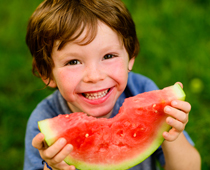[ ] N [ ] M [ ] U [ ] W
4. Here is a snake. Which of these is the upper case letter for ‘s’?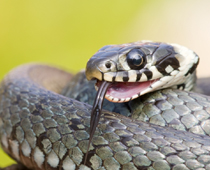[ ] G [ ] K [ ] R [ ] S
5. Fingers are needed to write and type. Which of these is a lower case ‘F’?[ ] f [ ] l [ ] g [ ] n
6. X marks the spot for treasure on this map. Which of these is the lower case letter for ‘X’?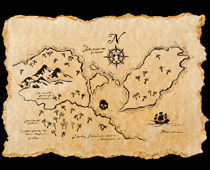[ ] v [ ] w [ ] m [ ] x
7. This is a picture of a valley. Which of these is the upper case letter for ‘v’?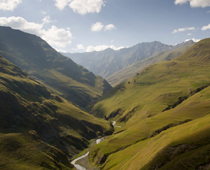[ ] W [ ] V [ ] N [ ] M
8. Perch begins with a capital ‘P’. Which of these letters is a lower case ‘P’?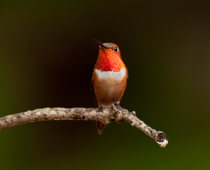[ ] d [ ] q [ ] p [ ] b
9. This is a picture of a zigzag. Which of these letters is an upper case ‘z’?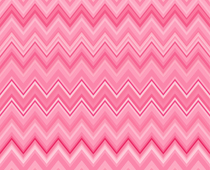[ ] X [ ] V [ ] W [ ] Z
10. The word ball should only have a capital letter when starting a sentence. Which of these is the upper case letter for ‘b’?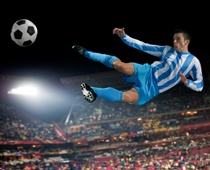[ ] P [ ] D [ ] G [ ] BKS1 English Quiz - Alphabet - Upper and Lower Case Letters 2 (Answers)
1. Egg begins with ‘E’ but should only be capitalized when at the beginning of a sentence. Which of these is the lower case letter for ‘E’?[ ] f [x] e [ ] a [ ] n
If you turn an egg on its side, it looks like the shape of a lower case ‘e’ but with a tail beneath it.
2. Jump begins with a capital 'J' when at the beginning of a sentence. Which of these is the lower case letter for ‘J’?[ ] l [x] j [ ] t [ ] s
The capital 'J' sits on top of the line but the lower case letter jumps down a little bit so that the line goes halfway through him - this will then help you join him up to other lower case letters.
3. The word nibble begins with ‘n’. Which of these is the upper case letter for ‘n’?[x] N [ ] M [ ] U [ ] W
An upper case 'N' has three straight lines, two are standing upright and one has fallen to the left. 'N' looks like 'M' but one of the lines has been nibbled away.
4. Here is a snake. Which of these is the upper case letter for ‘s’?[ ] G [ ] K [ ] R [x] S
'S' looks the same when written as an upper and lower case letter, the only difference is the size. It also looks wiggly like a snake.
5. Fingers are needed to write and type. Which of these is a lower case ‘F’?[x] f [ ] l [ ] g [ ] n
'Fingers' begins with an ‘f’ and the technical word for fingers is phalanges, which also begins with a ‘f’ sound.
6. X marks the spot for treasure on this map. Which of these is the lower case letter for ‘X’?[ ] v [ ] w [ ] m [x] x
There are only around 120 words that begin with ‘x’ in the English dictionary. The letter ‘x’ is also used on treasure maps and to represent kisses at the end of a birthday card!
7. This is a picture of a valley. Which of these is the upper case letter for ‘v’?[ ] W [x] V [ ] N [ ] M
Valleys look like the letter ‘v’. Often at the bottom of a valley is a river.
8. Perch begins with a capital ‘P’. Which of these letters is a lower case ‘P’?[ ] d [ ] q [x] p [ ] b
When 'P' is a capital, he stands on the line but when he is a lower case letter, he perches his belly on the line.
9. This is a picture of a zigzag. Which of these letters is an upper case ‘z’?[ ] X [ ] V [ ] W [x] Z
Zigzags make the same shape as a ‘z’ and the lower and upper case look the same but are different in size.
10. The word ball should only have a capital letter when starting a sentence. Which of these is the upper case letter for ‘b’?[ ] P [ ] D [ ] G [x] B
A capital 'B' looks like it has two balls stuck to the side of it.